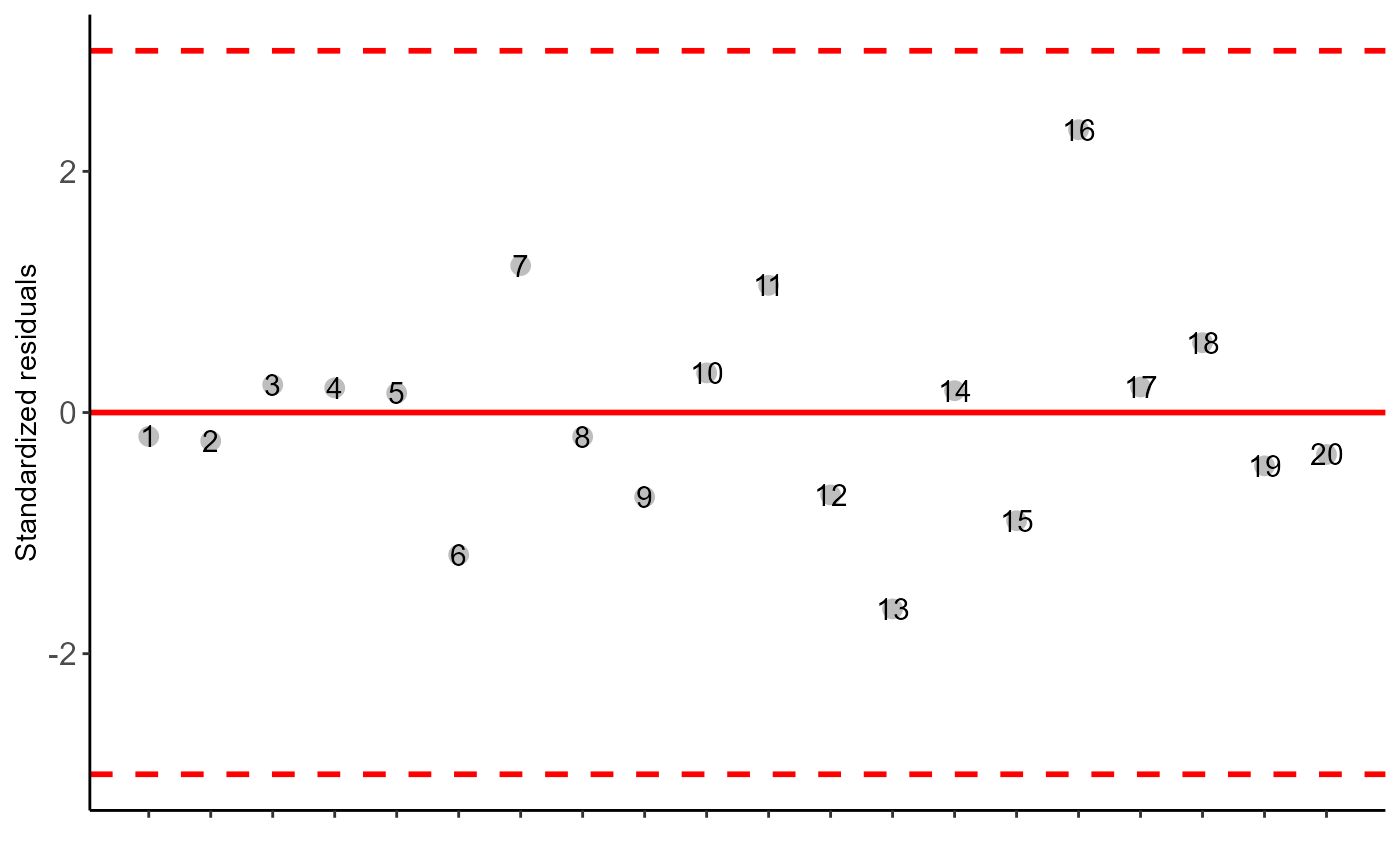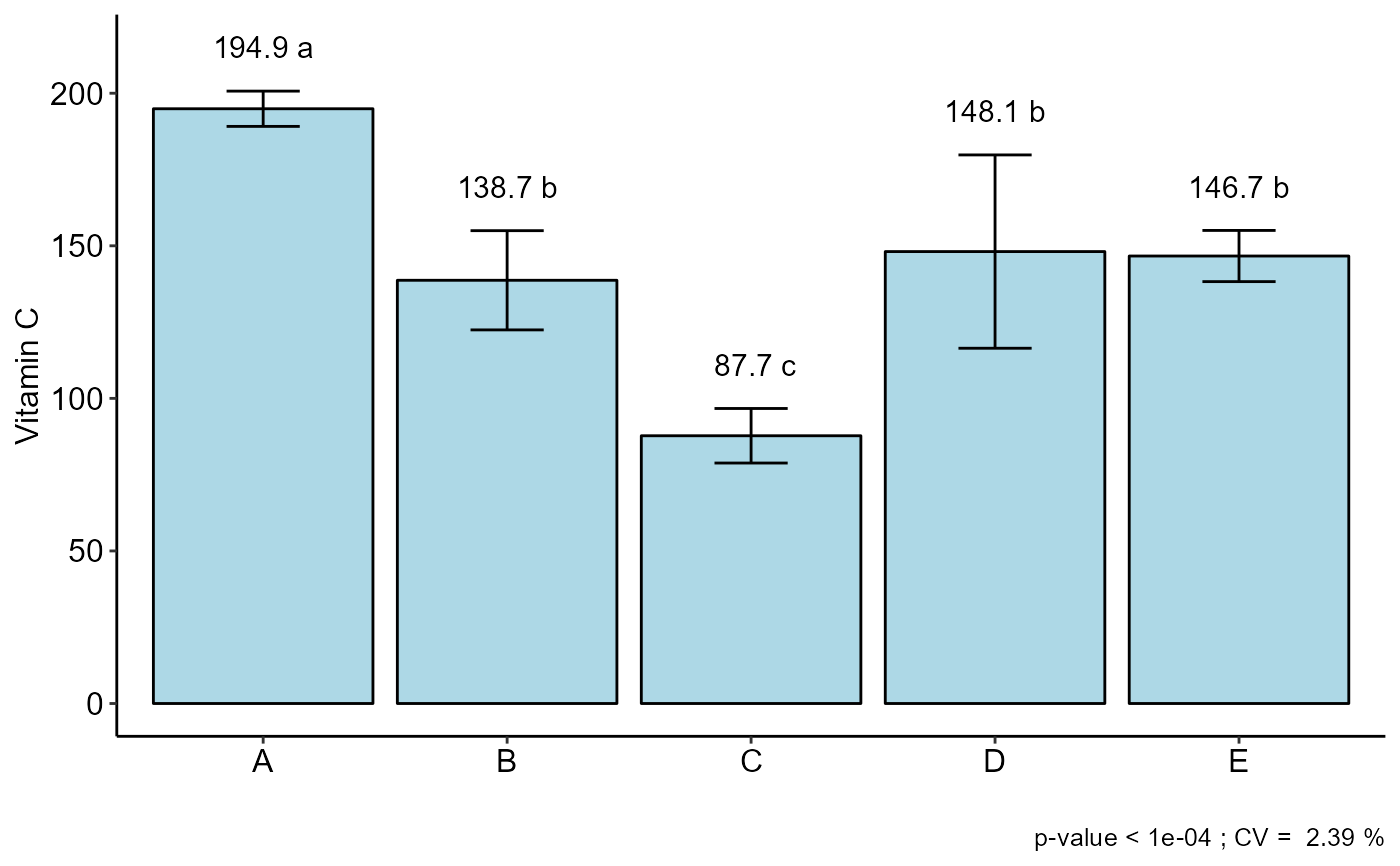Statistical analysis of experiments conducted in a completely randomized and balanced design with a factor considering the fixed model. The function presents the option to use non-parametric method or transform the dataset.

DIC(
trat,
response,
norm = "sw",
homog = "bt",
alpha.f = 0.05,
alpha.t = 0.05,
quali = TRUE,
mcomp = "tukey",
grau = 1,
transf = 1,
constant = 0,
test = "parametric",
mcompNP = "LSD",
geom = "bar",
theme = theme_classic(),
ylab = "Response",
sup = NA,
CV = TRUE,
xlab = "",
fill = "lightblue",
angle = 0,
family = "sans",
textsize = 12,
labelsize = 4,
dec = 3,
width.column = NULL,
width.bar = 0.3,
errorbar = TRUE,
posi = "top",
point = "mean_sd",
angle.label = 0,
ylim = NA
)

## Arguments

trat

Numerical or complex vector with treatments

response

Numerical vector containing the response of the experiment.

norm

Error normality test (default is Shapiro-Wilk)

homog

Homogeneity test of variances (default is Bartlett)

alpha.f

Level of significance of the F test (default is 0.05)

alpha.t

Significance level of the multiple comparison test (default is 0.05)

quali

Defines whether the factor is quantitative or qualitative (default is qualitative)

mcomp

Multiple comparison test (Tukey (default), LSD, Scott-Knott and Duncan)

grau

Degree of polynomial in case of quantitative factor (default is 1)

transf

Applies data transformation (default is 1; for log consider 0, angular for angular transformation)

constant

Add a constant for transformation (enter value)

test

"parametric" - Parametric test or "noparametric" - non-parametric test

mcompNP

Multiple comparison test (LSD (default) or dunn)

Method for adjusting p values for Kruskal-Wallis ("none","holm","hommel", "hochberg", "bonferroni", "BH", "BY", "fdr")

geom

Graph type (columns, boxes or segments)

theme

ggplot2 theme (default is theme_classic())

ylab

Variable response name (Accepts the expression() function)

sup

Number of units above the standard deviation or average bar on the graph

CV

Plotting the coefficient of variation and p-value of Anova (default is TRUE)

xlab

Treatments name (Accepts the expression() function)

fill

Defines chart color (to generate different colors for different treatments, define fill = "trat")

angle

x-axis scale text rotation

family

Font family

textsize

Font size

labelsize

Label size

dec

Number of cells

width.column

Width column if geom="bar"

width.bar

Width errorbar

Plot the average value on the graph (default is TRUE)

errorbar

Plot the standard deviation bar on the graph (In the case of a segment and column graph) - default is TRUE

posi

Legend position

point

Defines whether to plot mean ("mean"), mean with standard deviation ("mean_sd" - default) or mean with standard error ("mean_se"). For quali=FALSE or quali=TRUE. For parametric test it is possible to plot the square root of QMres (mean_qmres)

angle.label

label angle

ylim

Define a numerical sequence referring to the y scale. You can use a vector or the seq command.

## Value

The table of analysis of variance, the test of normality of errors (Shapiro-Wilk ("sw"), Lilliefors ("li"), Anderson-Darling ("ad"), Cramer-von Mises ("cvm"), Pearson ("pearson") and Shapiro-Francia ("sf")), the test of homogeneity of variances (Bartlett ("bt") or Levene ("levene")), the test of independence of Durbin-Watson errors, the test of multiple comparisons (Tukey ("tukey"), LSD ("lsd"), Scott-Knott ("sk") or Duncan ("duncan")) or adjustment of regression models up to grade 3 polynomial, in the case of quantitative treatments. Non-parametric analysis can be used by the Kruskal-Wallis test. The column, segment or box chart for qualitative treatments is also returned. The function also returns a standardized residual plot.

## Note

Enable ggplot2 package to change theme argument.

The ordering of the graph is according to the sequence in which the factor levels are arranged in the data sheet. The bars of the column and segment graphs are standard deviation.

Post hoc test in nonparametric is using the criterium Fisher's least significant difference (p-adj="holm").

CV and p-value of the graph indicate coefficient of variation and p-value of the F test of the analysis of variance.

In the final output when transformation (transf argument) is different from 1, the columns resp and respo in the mean test are returned, indicating transformed and non-transformed mean, respectively.

## Author

Gabriel Danilo Shimizu, shimizu@uel.br

Leandro Simoes Azeredo Goncalves

Rodrigo Yudi Palhaci Marubayashi

## Examples

library(AgroR)
data(pomegranate)

with(pomegranate, DIC(trat, WL, ylab = "Weight loss (%)")) # tukey
#>
#> -----------------------------------------------------------------
#> Normality of errors
#> -----------------------------------------------------------------
#>                          Method Statistic   p.value
#>  Shapiro-Wilk normality test(W) 0.9448293 0.2087967
#>
#> As the calculated p-value is greater than the 5% significance level, hypothesis H0 is not rejected. Therefore, errors can be considered normal
#>
#> -----------------------------------------------------------------
#> Homogeneity of Variances
#> -----------------------------------------------------------------
#>                               Method Statistic   p.value
#>  Bartlett test(Bartlett's K-squared)  8.568274 0.1275737
#>
#> As the calculated p-value is greater than the 5% significance level,hypothesis H0 is not rejected. Therefore, the variances can be considered homogeneous
#>
#> -----------------------------------------------------------------
#> Independence from errors
#> -----------------------------------------------------------------
#>                  Method Statistic   p.value
#>  Durbin-Watson test(DW)  2.104821 0.1924474
#>
#> As the calculated p-value is greater than the 5% significance level, hypothesis H0 is not rejected. Therefore, errors can be considered independent
#>
#> -----------------------------------------------------------------
#> -----------------------------------------------------------------
#>
#> CV (%) =  10.84
#> MStrat/MST =  0.92
#> Mean =  2.2596
#> Median =  2.225
#> Possible outliers =  No discrepant point
#>
#> -----------------------------------------------------------------
#> Analysis of Variance
#> -----------------------------------------------------------------
#>           Df   Sum Sq    Mean.Sq  F value        Pr(F)
#> trat       5 3.692121 0.73842417 12.31191 2.723541e-05
#> Residuals 18 1.079575 0.05997639
#>
#>
#> As the calculated p-value, it is less than the 5% significance level.The hypothesis H0 of equality of means is rejected. Therefore, at least two treatments differ
#>
#>
#> -----------------------------------------------------------------
#> Multiple Comparison Test: Tukey HSD
#> -----------------------------------------------------------------
#>      resp groups
#> T5 2.6375      a
#> T4 2.6200      a
#> T3 2.6175      a
#> T6 2.1625     ab
#> T1 1.8425      b
#> T2 1.6775      b
#>
#>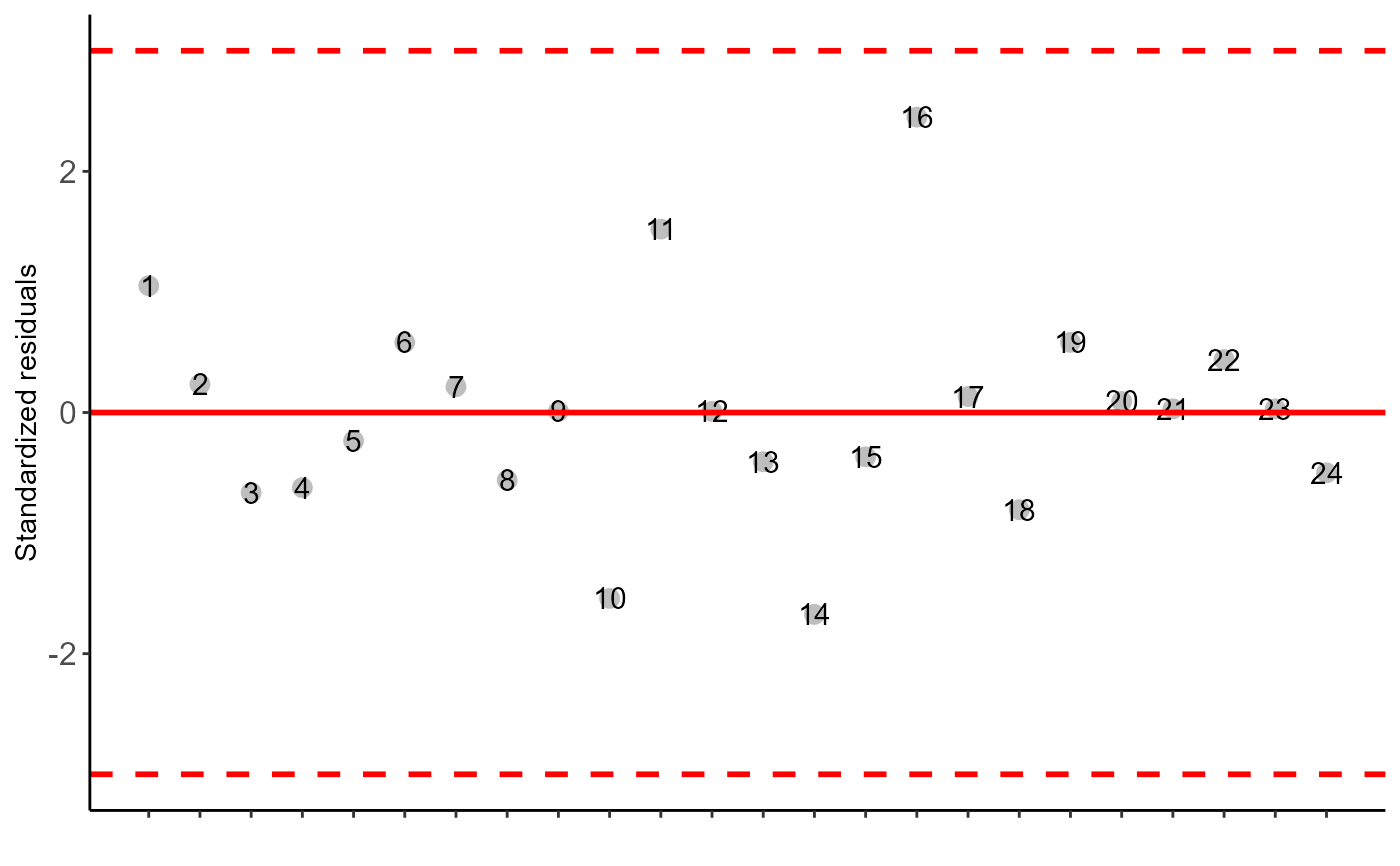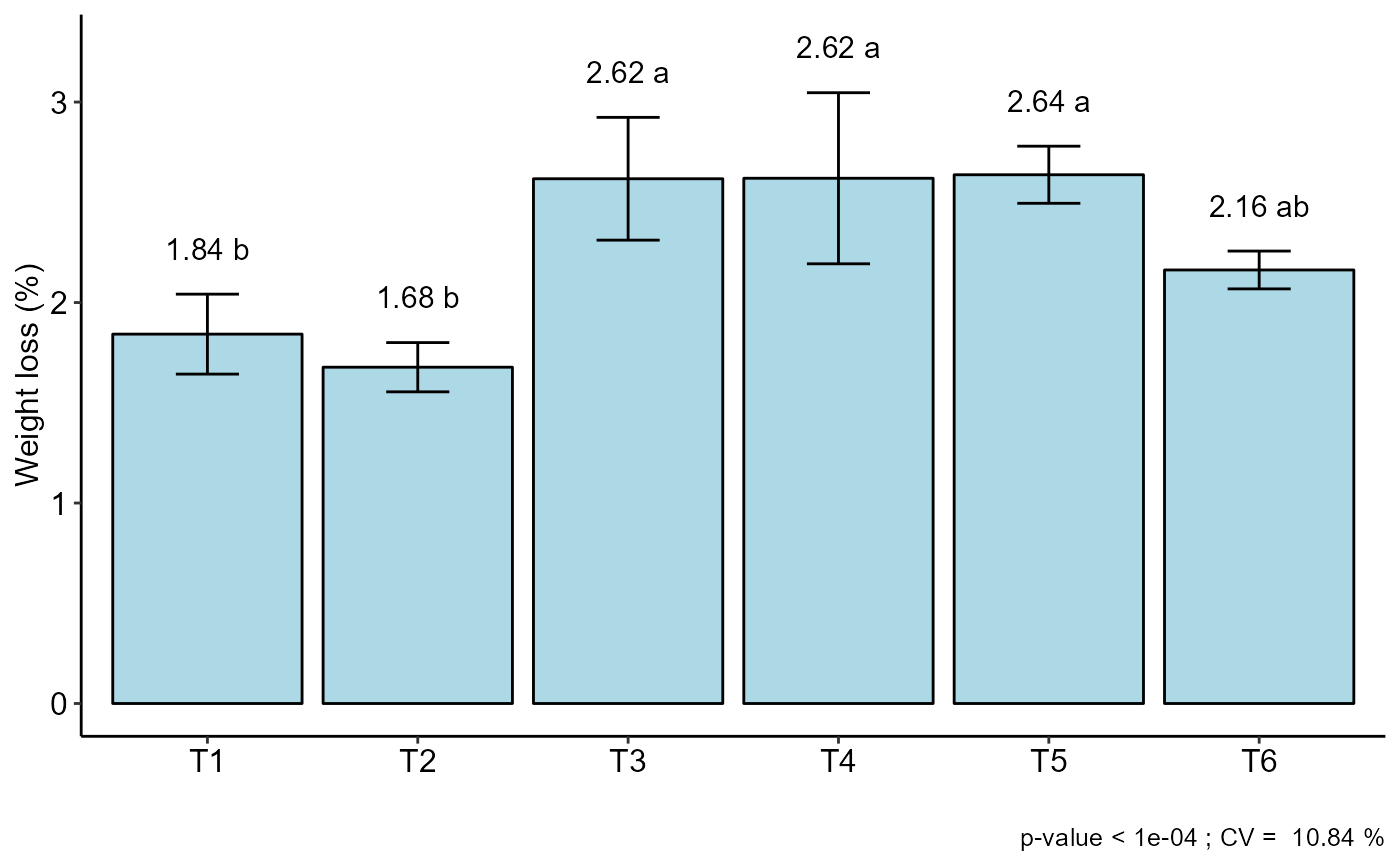with(pomegranate, DIC(trat, WL, mcomp = "sk", ylab = "Weight loss (%)"))
#>
#> -----------------------------------------------------------------
#> Normality of errors
#> -----------------------------------------------------------------
#>                          Method Statistic   p.value
#>  Shapiro-Wilk normality test(W) 0.9448293 0.2087967
#>
#> As the calculated p-value is greater than the 5% significance level, hypothesis H0 is not rejected. Therefore, errors can be considered normal
#>
#> -----------------------------------------------------------------
#> Homogeneity of Variances
#> -----------------------------------------------------------------
#>                               Method Statistic   p.value
#>  Bartlett test(Bartlett's K-squared)  8.568274 0.1275737
#>
#> As the calculated p-value is greater than the 5% significance level,hypothesis H0 is not rejected. Therefore, the variances can be considered homogeneous
#>
#> -----------------------------------------------------------------
#> Independence from errors
#> -----------------------------------------------------------------
#>                  Method Statistic   p.value
#>  Durbin-Watson test(DW)  2.104821 0.1924474
#>
#> As the calculated p-value is greater than the 5% significance level, hypothesis H0 is not rejected. Therefore, errors can be considered independent
#>
#> -----------------------------------------------------------------
#> -----------------------------------------------------------------
#>
#> CV (%) =  10.84
#> MStrat/MST =  0.92
#> Mean =  2.2596
#> Median =  2.225
#> Possible outliers =  No discrepant point
#>
#> -----------------------------------------------------------------
#> Analysis of Variance
#> -----------------------------------------------------------------
#>           Df   Sum Sq    Mean.Sq  F value        Pr(F)
#> trat       5 3.692121 0.73842417 12.31191 2.723541e-05
#> Residuals 18 1.079575 0.05997639
#>
#>
#> As the calculated p-value, it is less than the 5% significance level.The hypothesis H0 of equality of means is rejected. Therefore, at least two treatments differ
#>
#>
#> -----------------------------------------------------------------
#> Multiple Comparison Test: Scott-Knott
#> -----------------------------------------------------------------
#>      resp groups
#> T5 2.6375      a
#> T4 2.6200      a
#> T3 2.6175      a
#> T6 2.1625      b
#> T1 1.8425      c
#> T2 1.6775      c
#>
#>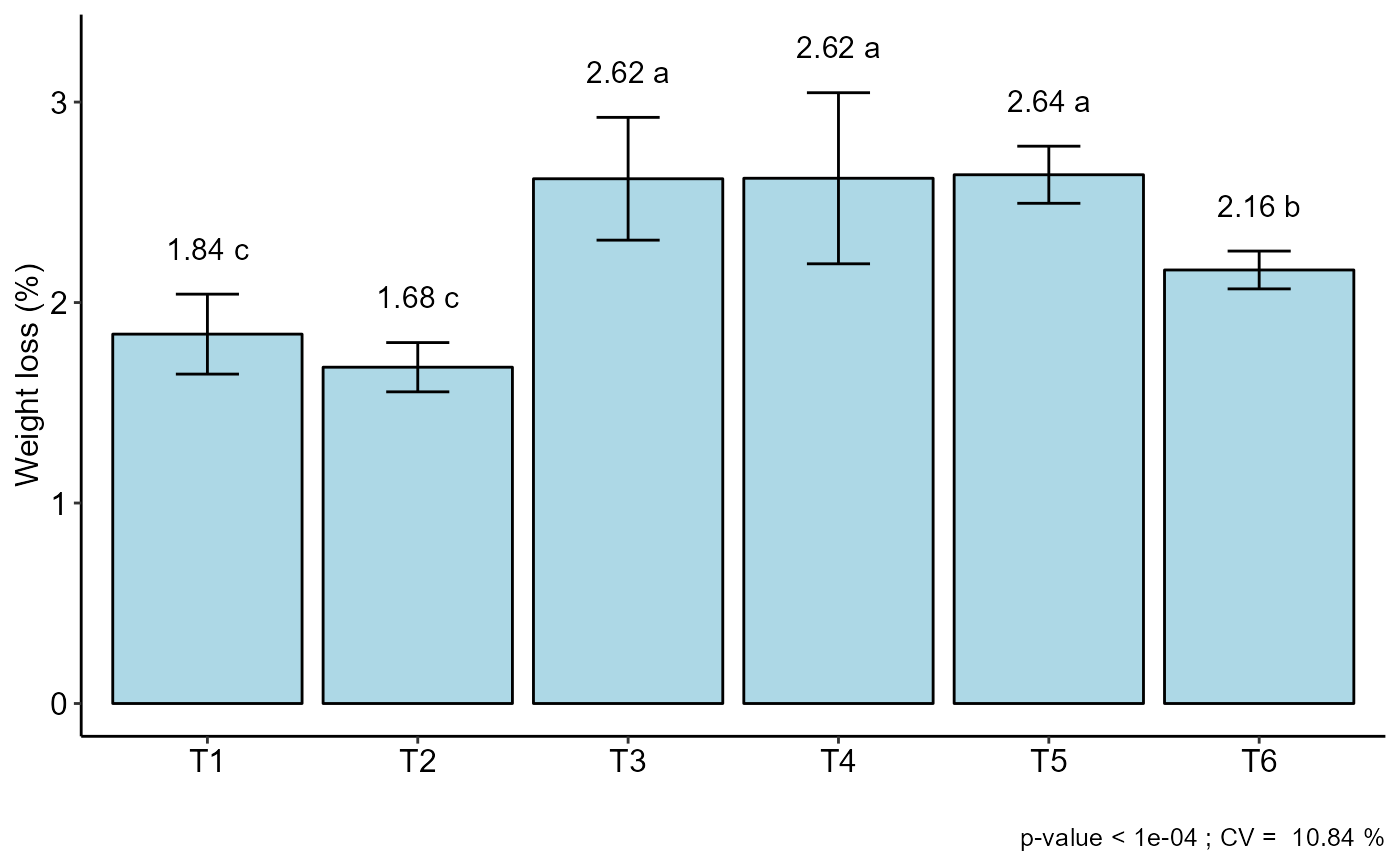with(pomegranate, DIC(trat, WL, mcomp = "duncan", ylab = "Weight loss (%)"))
#>
#> -----------------------------------------------------------------
#> Normality of errors
#> -----------------------------------------------------------------
#>                          Method Statistic   p.value
#>  Shapiro-Wilk normality test(W) 0.9448293 0.2087967
#>
#> As the calculated p-value is greater than the 5% significance level, hypothesis H0 is not rejected. Therefore, errors can be considered normal
#>
#> -----------------------------------------------------------------
#> Homogeneity of Variances
#> -----------------------------------------------------------------
#>                               Method Statistic   p.value
#>  Bartlett test(Bartlett's K-squared)  8.568274 0.1275737
#>
#> As the calculated p-value is greater than the 5% significance level,hypothesis H0 is not rejected. Therefore, the variances can be considered homogeneous
#>
#> -----------------------------------------------------------------
#> Independence from errors
#> -----------------------------------------------------------------
#>                  Method Statistic   p.value
#>  Durbin-Watson test(DW)  2.104821 0.1924474
#>
#> As the calculated p-value is greater than the 5% significance level, hypothesis H0 is not rejected. Therefore, errors can be considered independent
#>
#> -----------------------------------------------------------------
#> -----------------------------------------------------------------
#>
#> CV (%) =  10.84
#> MStrat/MST =  0.92
#> Mean =  2.2596
#> Median =  2.225
#> Possible outliers =  No discrepant point
#>
#> -----------------------------------------------------------------
#> Analysis of Variance
#> -----------------------------------------------------------------
#>           Df   Sum Sq    Mean.Sq  F value        Pr(F)
#> trat       5 3.692121 0.73842417 12.31191 2.723541e-05
#> Residuals 18 1.079575 0.05997639
#>
#>
#> As the calculated p-value, it is less than the 5% significance level.The hypothesis H0 of equality of means is rejected. Therefore, at least two treatments differ
#>
#>
#> -----------------------------------------------------------------
#> Multiple Comparison Test: Duncan
#> -----------------------------------------------------------------
#>      resp groups
#> T5 2.6375      a
#> T4 2.6200      a
#> T3 2.6175      a
#> T6 2.1625      b
#> T1 1.8425     bc
#> T2 1.6775      c
#>
#>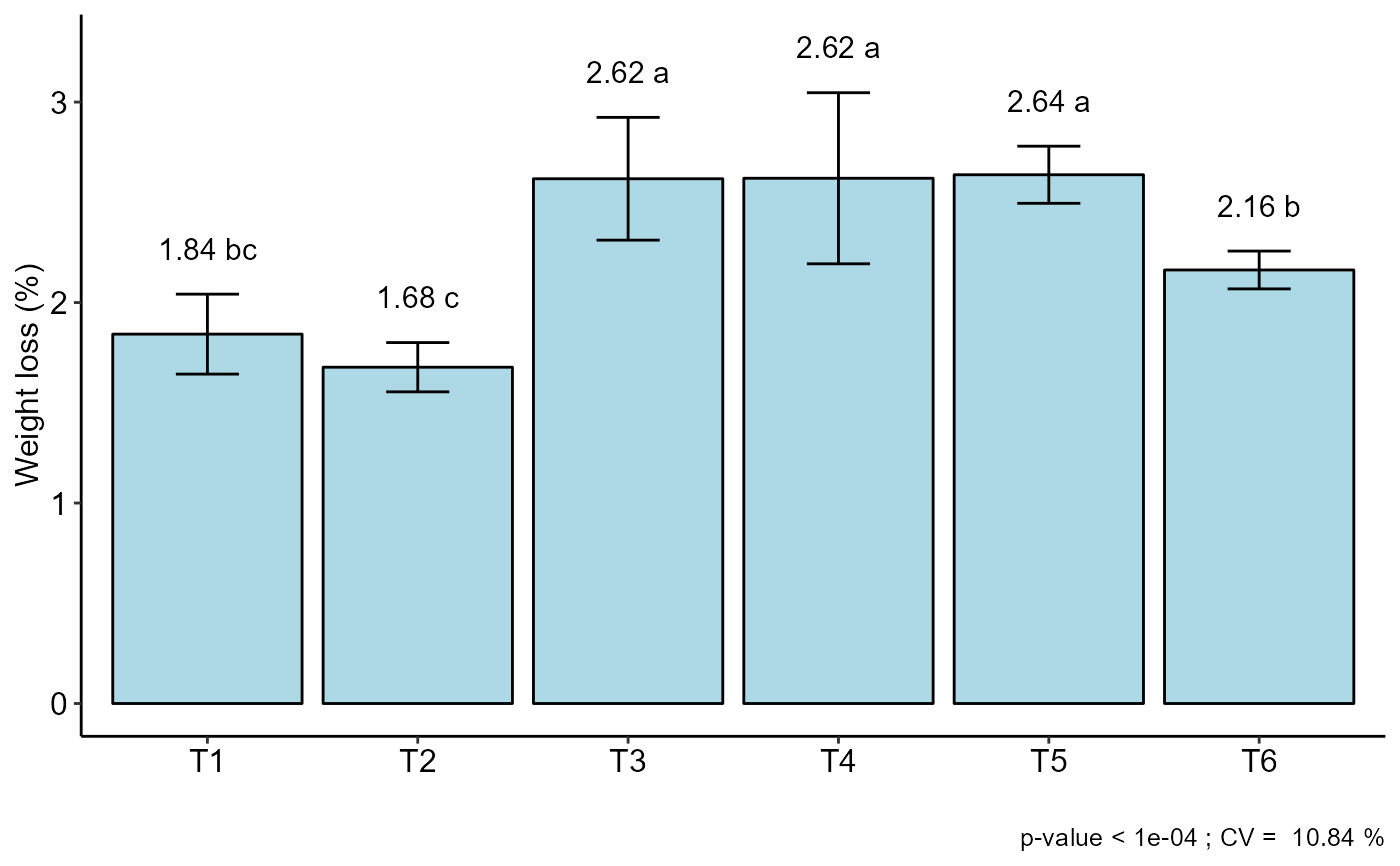#=============================
# Kruskal-Wallis
#=============================
with(pomegranate, DIC(trat, WL, test = "noparametric", ylab = "Weight loss (%)"))
#>
#>
#> -----------------------------------------------------------------
#> Statistics
#> -----------------------------------------------------------------
#>      Chisq     p.chisq
#>   18.75631 0.002133687
#>
#>
#> -----------------------------------------------------------------
#> Parameters
#> -----------------------------------------------------------------
#>             test p.ajusted name.t ntr alpha
#>   Kruskal-Wallis      holm   trat   6  0.05
#>
#>
#> -----------------------------------------------------------------
#> Multiple Comparison Test: LSD
#> -----------------------------------------------------------------
#>      Mean         SD  Rank Groups
#> T1 1.8425 0.19939492  5.75      c
#> T2 1.6775 0.12284814  3.50      c
#> T3 2.6175 0.30619983 18.25     ab
#> T4 2.6200 0.42669271 17.25     ab
#> T5 2.6375 0.14244882 19.50      a
#> T6 2.1625 0.09429563 10.75     bc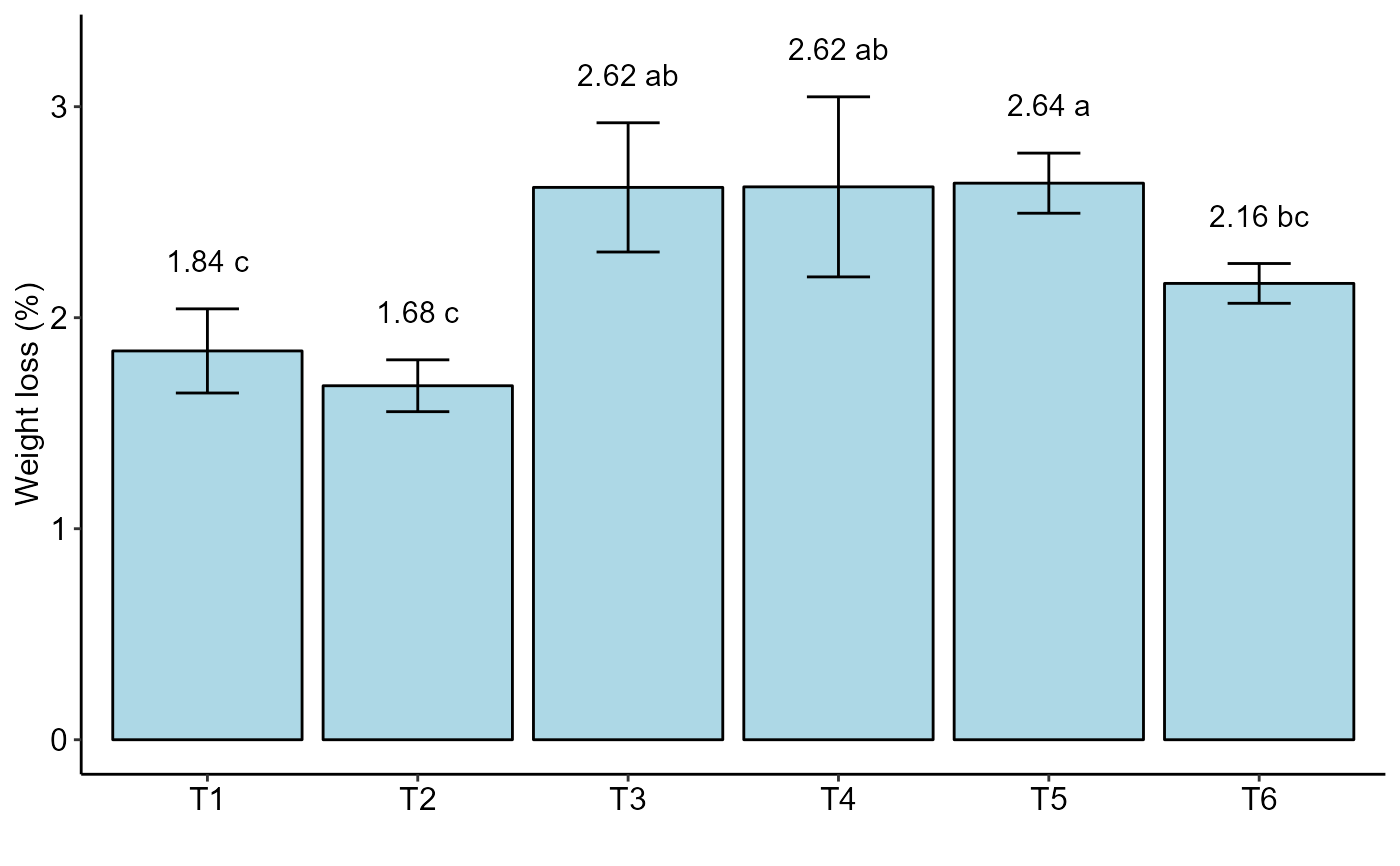#=============================
# chart type
#=============================
with(pomegranate, DIC(trat, WL, geom="point", ylab = "Weight loss (%)"))
#>
#> -----------------------------------------------------------------
#> Normality of errors
#> -----------------------------------------------------------------
#>                          Method Statistic   p.value
#>  Shapiro-Wilk normality test(W) 0.9448293 0.2087967
#>
#> As the calculated p-value is greater than the 5% significance level, hypothesis H0 is not rejected. Therefore, errors can be considered normal
#>
#> -----------------------------------------------------------------
#> Homogeneity of Variances
#> -----------------------------------------------------------------
#>                               Method Statistic   p.value
#>  Bartlett test(Bartlett's K-squared)  8.568274 0.1275737
#>
#> As the calculated p-value is greater than the 5% significance level,hypothesis H0 is not rejected. Therefore, the variances can be considered homogeneous
#>
#> -----------------------------------------------------------------
#> Independence from errors
#> -----------------------------------------------------------------
#>                  Method Statistic   p.value
#>  Durbin-Watson test(DW)  2.104821 0.1924474
#>
#> As the calculated p-value is greater than the 5% significance level, hypothesis H0 is not rejected. Therefore, errors can be considered independent
#>
#> -----------------------------------------------------------------
#> -----------------------------------------------------------------
#>
#> CV (%) =  10.84
#> MStrat/MST =  0.92
#> Mean =  2.2596
#> Median =  2.225
#> Possible outliers =  No discrepant point
#>
#> -----------------------------------------------------------------
#> Analysis of Variance
#> -----------------------------------------------------------------
#>           Df   Sum Sq    Mean.Sq  F value        Pr(F)
#> trat       5 3.692121 0.73842417 12.31191 2.723541e-05
#> Residuals 18 1.079575 0.05997639
#>
#>
#> As the calculated p-value, it is less than the 5% significance level.The hypothesis H0 of equality of means is rejected. Therefore, at least two treatments differ
#>
#>
#> -----------------------------------------------------------------
#> Multiple Comparison Test: Tukey HSD
#> -----------------------------------------------------------------
#>      resp groups
#> T5 2.6375      a
#> T4 2.6200      a
#> T3 2.6175      a
#> T6 2.1625     ab
#> T1 1.8425      b
#> T2 1.6775      b
#>
#>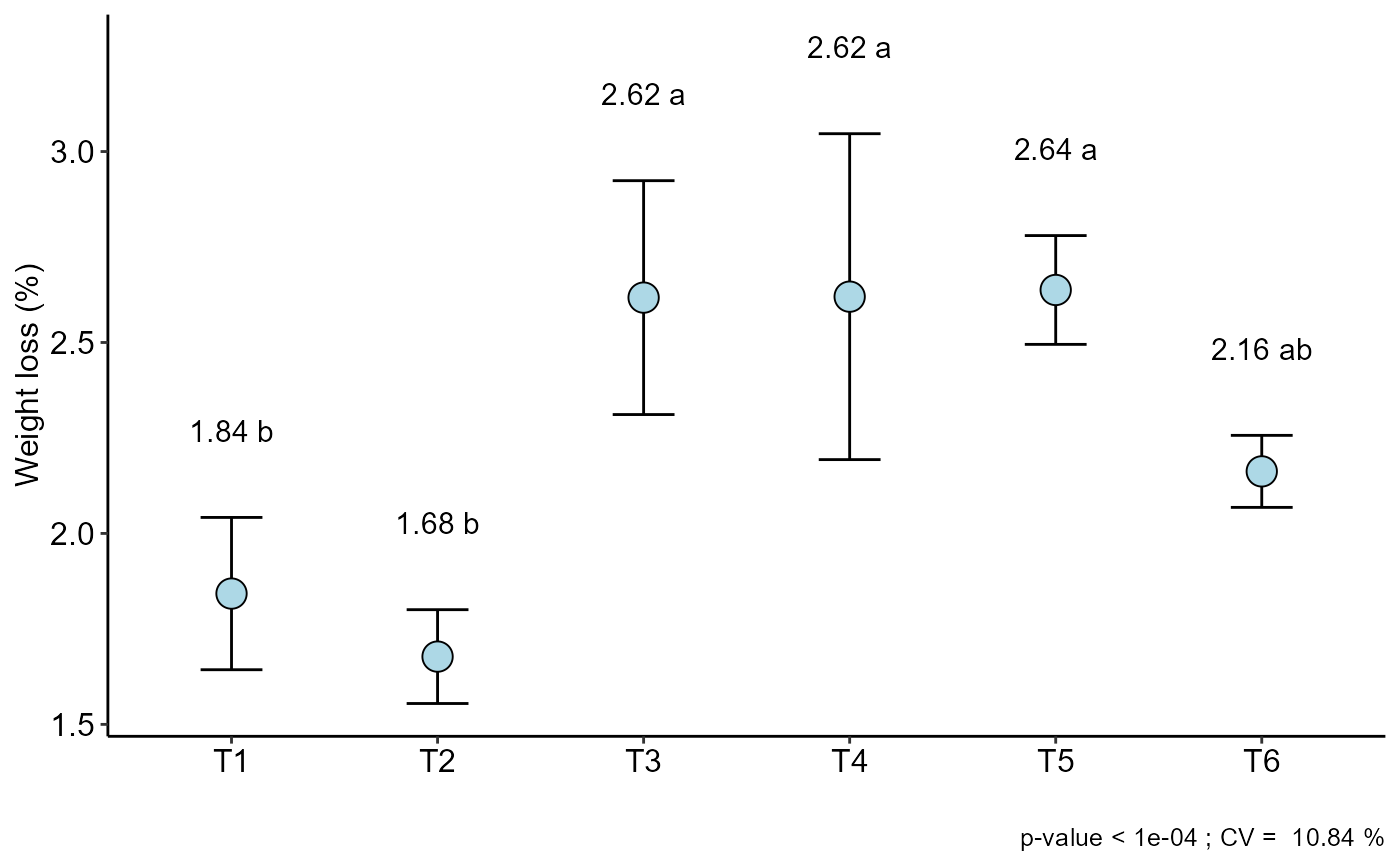with(pomegranate, DIC(trat, WL, ylab = "Weight loss (%)", xlab="Treatments"))
#>
#> -----------------------------------------------------------------
#> Normality of errors
#> -----------------------------------------------------------------
#>                          Method Statistic   p.value
#>  Shapiro-Wilk normality test(W) 0.9448293 0.2087967
#>
#> As the calculated p-value is greater than the 5% significance level, hypothesis H0 is not rejected. Therefore, errors can be considered normal
#>
#> -----------------------------------------------------------------
#> Homogeneity of Variances
#> -----------------------------------------------------------------
#>                               Method Statistic   p.value
#>  Bartlett test(Bartlett's K-squared)  8.568274 0.1275737
#>
#> As the calculated p-value is greater than the 5% significance level,hypothesis H0 is not rejected. Therefore, the variances can be considered homogeneous
#>
#> -----------------------------------------------------------------
#> Independence from errors
#> -----------------------------------------------------------------
#>                  Method Statistic   p.value
#>  Durbin-Watson test(DW)  2.104821 0.1924474
#>
#> As the calculated p-value is greater than the 5% significance level, hypothesis H0 is not rejected. Therefore, errors can be considered independent
#>
#> -----------------------------------------------------------------
#> -----------------------------------------------------------------
#>
#> CV (%) =  10.84
#> MStrat/MST =  0.92
#> Mean =  2.2596
#> Median =  2.225
#> Possible outliers =  No discrepant point
#>
#> -----------------------------------------------------------------
#> Analysis of Variance
#> -----------------------------------------------------------------
#>           Df   Sum Sq    Mean.Sq  F value        Pr(F)
#> trat       5 3.692121 0.73842417 12.31191 2.723541e-05
#> Residuals 18 1.079575 0.05997639
#>
#>
#> As the calculated p-value, it is less than the 5% significance level.The hypothesis H0 of equality of means is rejected. Therefore, at least two treatments differ
#>
#>
#> -----------------------------------------------------------------
#> Multiple Comparison Test: Tukey HSD
#> -----------------------------------------------------------------
#>      resp groups
#> T5 2.6375      a
#> T4 2.6200      a
#> T3 2.6175      a
#> T6 2.1625     ab
#> T1 1.8425      b
#> T2 1.6775      b
#>
#>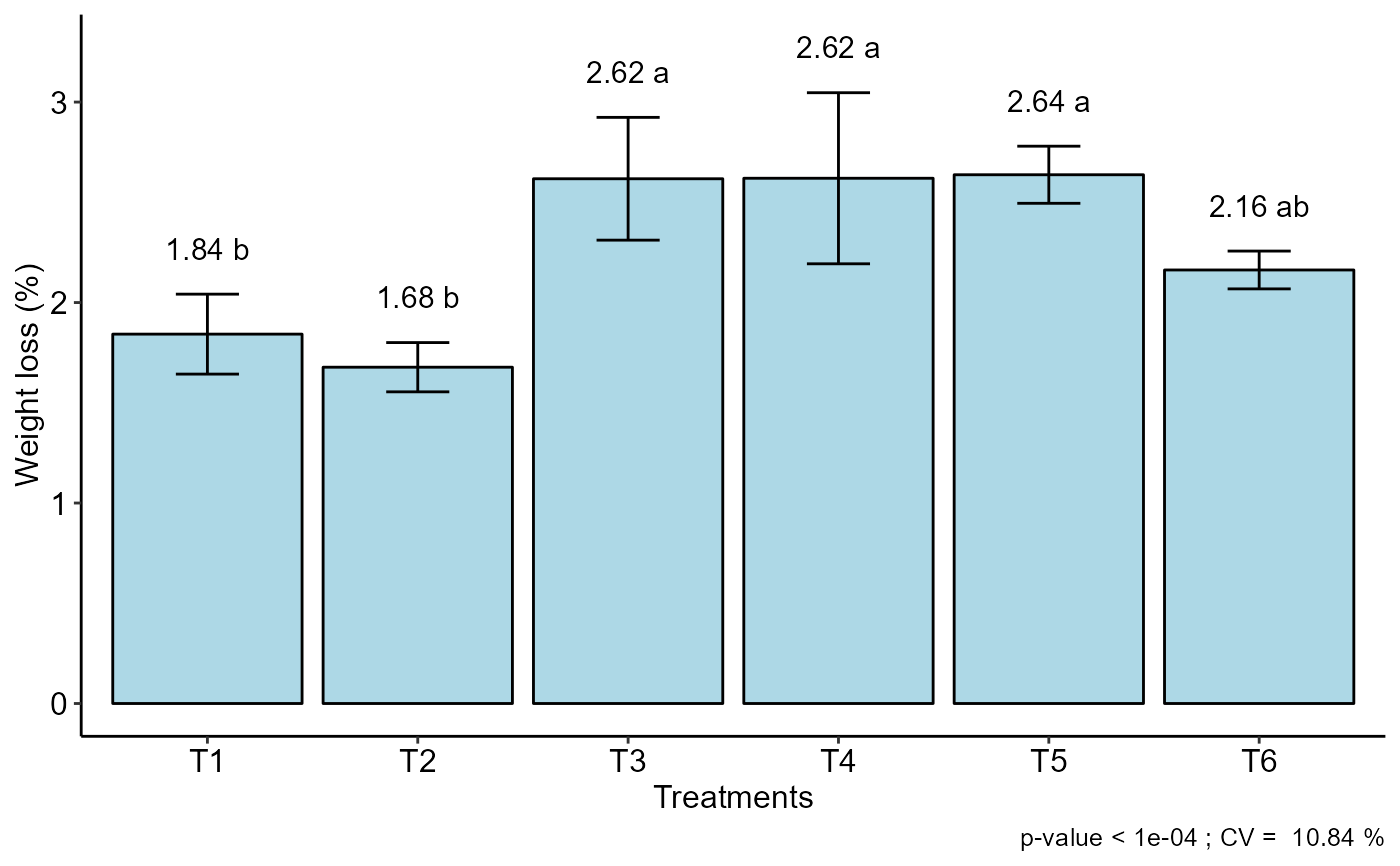#=============================
# quantitative factor
#=============================
data("phao")
with(phao, DIC(dose,comp,quali=FALSE,grau=2,
xlab = expression("Dose"~(g~vase^-1)),
ylab="Leaf length (cm)"))
#>
#> -----------------------------------------------------------------
#> Normality of errors
#> -----------------------------------------------------------------
#>                          Method Statistic   p.value
#>  Shapiro-Wilk normality test(W) 0.9647717 0.5174008
#>
#> As the calculated p-value is greater than the 5% significance level, hypothesis H0 is not rejected. Therefore, errors can be considered normal
#>
#> -----------------------------------------------------------------
#> Homogeneity of Variances
#> -----------------------------------------------------------------
#>                               Method Statistic   p.value
#>  Bartlett test(Bartlett's K-squared)  4.428915 0.3510598
#>
#> As the calculated p-value is greater than the 5% significance level,hypothesis H0 is not rejected. Therefore, the variances can be considered homogeneous
#>
#> -----------------------------------------------------------------
#> Independence from errors
#> -----------------------------------------------------------------
#>                  Method Statistic    p.value
#>  Durbin-Watson test(DW)  1.801827 0.08064338
#>
#> As the calculated p-value is greater than the 5% significance level, hypothesis H0 is not rejected. Therefore, errors can be considered independent
#>
#> -----------------------------------------------------------------
#> -----------------------------------------------------------------
#>
#> CV (%) =  11.71
#> MStrat/MST =  0.93
#> Mean =  14.436
#> Median =  15.3
#> Possible outliers =  No discrepant point
#>
#> -----------------------------------------------------------------
#> Analysis of Variance
#> -----------------------------------------------------------------
#>           Df   Sum Sq Mean.Sq  F value        Pr(F)
#> trat       4 145.8096 36.4524 12.76166 2.557884e-05
#> Residuals 20  57.1280  2.8564
#>
#>
#> As the calculated p-value, it is less than the 5% significance level.The hypothesis H0 of equality of means is rejected. Therefore, at least two treatments differ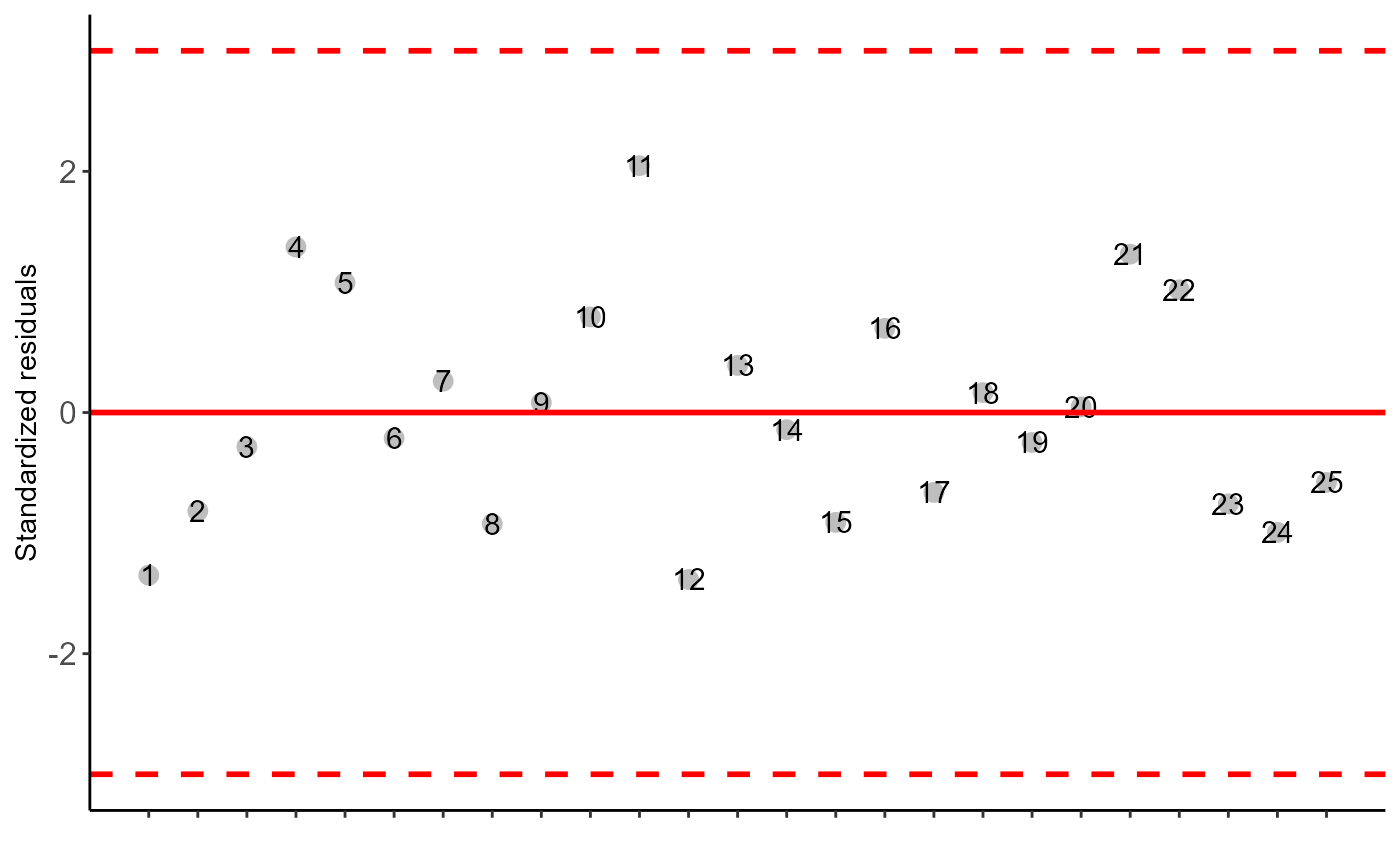#>
#>
#> -----------------------------------------------------------------
#> Regression
#> -----------------------------------------------------------------
#>
#> ----------------------------------------------------
#> Regression Models
#> ----------------------------------------------------
#>               Estimate Std. Error   t value     Pr(>|t|)
#> (Intercept) 10.2097143 0.68981140 14.800733 6.427099e-13
#> trat         2.8822857 0.40856781  7.054608 4.456995e-07
#> I(trat^2)   -0.3042857 0.04897332 -6.213296 2.971498e-06
#>
#> ----------------------------------------------------
#> Deviations from regression
#> ----------------------------------------------------
#>           Df        SSq         MSQ          F      p-value
#> Linear     1  40.140800  40.1408000 14.0529338 1.265122e-03
#> Quadratic  1 103.700571 103.7005714 36.3046392 6.852202e-06
#> Deviation  2   1.968229   0.9841143  0.3445296 7.126767e-01
#> Residual  20  57.128000   2.8564000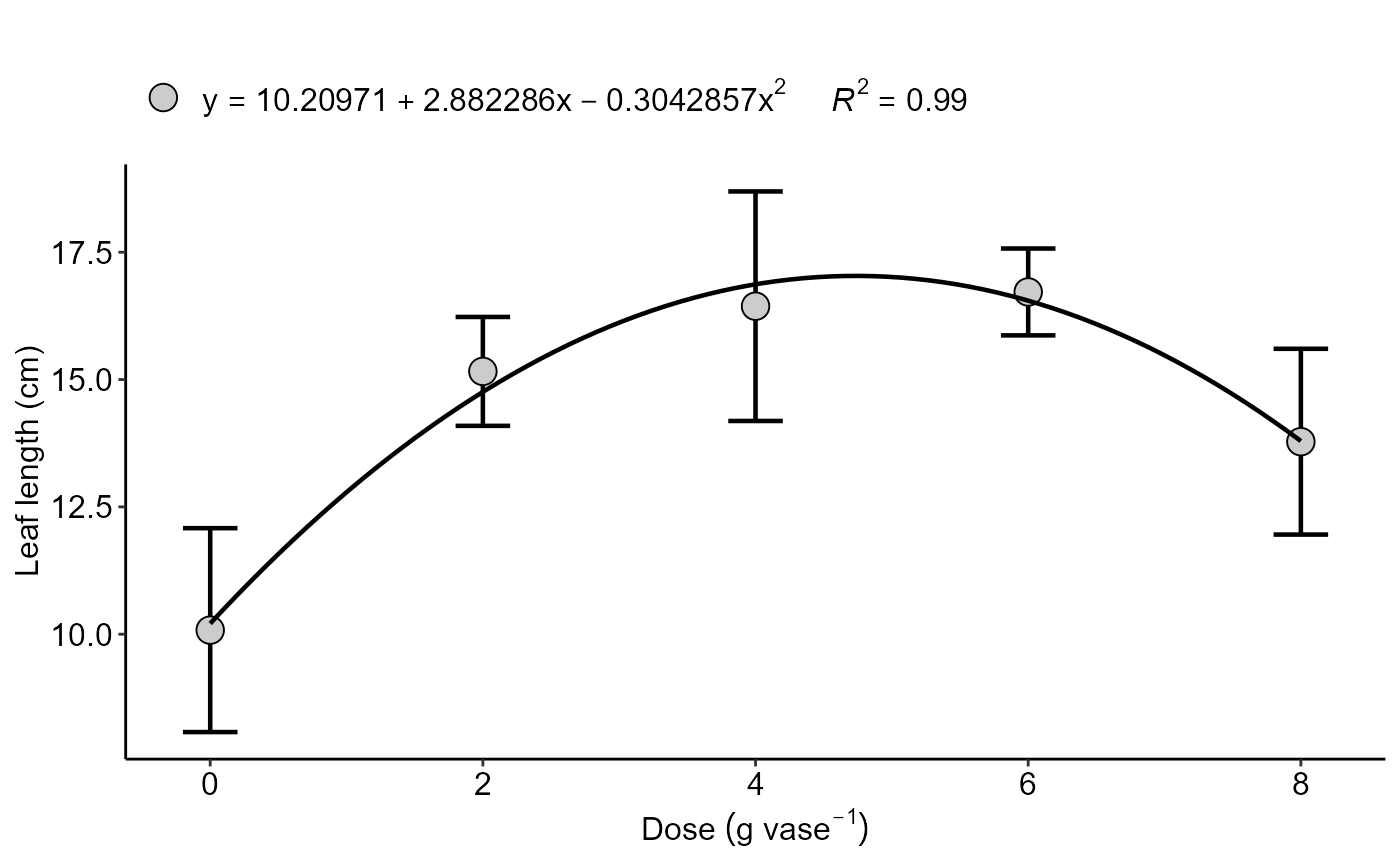#=============================
# data transformation
#=============================
data("pepper")
with(pepper, DIC(Acesso, VitC, transf = 0,ylab="Vitamin C"))
#>
#> -----------------------------------------------------------------
#> Normality of errors
#> -----------------------------------------------------------------
#>                          Method Statistic   p.value
#>  Shapiro-Wilk normality test(W) 0.9568114 0.4822784
#>
#> As the calculated p-value is greater than the 5% significance level, hypothesis H0 is not rejected. Therefore, errors can be considered normal
#>
#> -----------------------------------------------------------------
#> Homogeneity of Variances
#> -----------------------------------------------------------------
#>                               Method Statistic    p.value
#>  Bartlett test(Bartlett's K-squared)  9.166029 0.05708089
#>
#> As the calculated p-value is greater than the 5% significance level,hypothesis H0 is not rejected. Therefore, the variances can be considered homogeneous
#>
#> -----------------------------------------------------------------
#> Independence from errors
#> -----------------------------------------------------------------
#>                  Method Statistic   p.value
#>  Durbin-Watson test(DW)  2.415765 0.5054283
#>
#> As the calculated p-value is greater than the 5% significance level, hypothesis H0 is not rejected. Therefore, errors can be considered independent
#>
#> -----------------------------------------------------------------
#> -----------------------------------------------------------------
#>
#> CV (%) =  2.39
#> MStrat/MST =  0.96
#> Mean =  143.223
#> Median =  140.615
#> Possible outliers =  No discrepant point
#>
#> -----------------------------------------------------------------
#> Analysis of Variance
#> -----------------------------------------------------------------
#>           Df    Sum Sq    Mean.Sq  F value        Pr(F)
#> trat       4 1.3360870 0.33402175 23.98432 2.274302e-06
#> Residuals 15 0.2089001 0.01392667
#>
#>
#> As the calculated p-value, it is less than the 5% significance level.The hypothesis H0 of equality of means is rejected. Therefore, at least two treatments differ
#>
#>
#> -----------------------------------------------------------------
#> Multiple Comparison Test: Tukey HSD
#> -----------------------------------------------------------------
#>       resp groups    respO
#> A 5.272221      a 194.9125
#> E 4.986831      b 146.6500
#> D 4.981784      b 148.1050
#> B 4.927180      b 138.7000
#> C 4.470638      c  87.7475
#>
#>
#> NOTE: resp = transformed means; respO = averages without transforming
#>